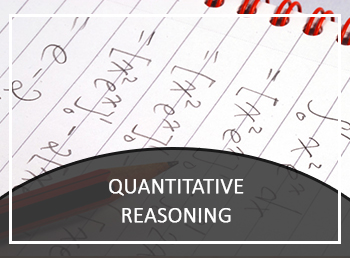# GMAT QUANTITATIVE REASONINGBundle Includes:
• Student Book
• Workbook

Product Format:  Print Product

Valid - Guaranteed

### Data Analyzation & Drawing Conclusions

The Quantitative Reasoning section of the GMAT exam consists of 31 multiple choice questions that are needed to be completed within 62 minutes. This part, in particular, tests your capability to cope with mathematical reasoning, solve quantitative problems and further on concluding it with graphical data interpretation.

### Different Question Types in the Quantitative Reasoning Section

Quantitative Reasoning consists of two types of questions:

• Problem Solving
• Data sufficiency

To be able to solve these questions a candidate must have foundational knowledge of basic arithmetic, algebra (elementary level) and fundamental concepts of geometry. If these things scared you, to your satisfaction I must admit that the answers depend on your logical and analytical senses rather than your mathematical skills. Also, you will be allowed to use a calculator during this section of the exam.

Following is the brief summary of what these questions involve:

### Problem Solving

• Tests how you use your logical and analytical skills to solve the quantitative problems.
• You are expected to select the best choice among given five after solving the problem.

### Data Sufficiency

• Data Sufficiency measure your skills to thoroughly examine data to find out which data is relevant to your problem and to what extent it can be used to solve the same problem.
• You are expected to determine whether the data are given in the problem (i.e. questions and two statements) is enough to answers the question validly using your knowledge of math and everyday facts.